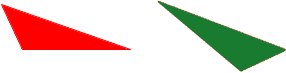SEARCH HOMEMath Central Quandaries & QueriesQuestion from tia: what is the name used for shapes that are exactly the same?Tia,

I think the word you are looking for is congruent.

Two geometric figures are called congruent if one can be transformed into the other by a combination of translations, rotations and reflections.

The two triangles below are congruent since you can pick up the red triangle, translate, rotate and reflect it and place it down exactly on top of the green triangle.It is common for mathematicians to consider geometrical figures to be the same if they are congruent. Outside mathematics I wouldn't say they are exactly the same, one is red and the other is green.

HarleyMath Central is supported by the University of Regina and The Pacific Institute for the Mathematical Sciences.